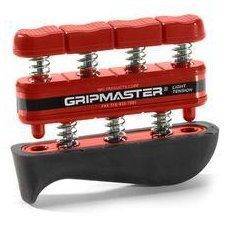# How much force is on each spring in this device?

pa5tabear
I have a grip strengthener which is rated at 7lbs. I'm wondering how that load is distributed among the seven springs. Would each spring offer 7lbs of resistance?If I'm compressing the device all the way, how much force am I really applying?

Mentor
Would each spring offer 7lbs of resistance?
Yes. The claim for that device is 7lbs per finger. Don't know how accurate that claim is.

pa5tabear
Yes. The claim for that device is 7lbs per finger. Don't know how accurate that claim is.

If it's 7lbs per finger, how does that distribute across the two layers of springs, one of which is three, and the other four?

I'm guessing that the "four layer" will offer more resistance than the "three layer" and that each spring will be degenerate within the layer.

If each finger is supposed to experience 7lbs of resistance, then the combination of the individual spring resisistance and the resistance from the three springs divided among four fingers should add up to 7lbs.

Last edited:
Mentor
If it's 7lbs per finger, how does that distribute across the two layers of springs, one of which is three, and the other four?

If I'm understanding the picture properly... Each of the four-layer springs is exerting a force of seven pounds on a single finger, and therefore also on the center bar. Thus the total downwards force on the center bar is 28 pounds.

This force is must be balanced by the upwards force of the three-layer springs, so the total force carried by these three springs is also 28 pounds (and it will be divided fairly evenly across the three if all four fingers are applying the same 7 pounds of force). 28/3 is about 9.3 pounds.

These three springs are therefore applying a total downwards force of 28 pounds on the bottommost bar. This is the force that must be balanced by the palm of your hand when you squeeze.

pa5tabear
If I'm understanding the picture properly... Each of the four-layer springs is exerting a force of seven pounds on a single finger, and therefore also on the center bar. Thus the total downwards force on the center bar is 28 pounds.

This force is must be balanced by the upwards force of the three-layer springs, so the total force carried by these three springs is also 28 pounds (and it will be divided fairly evenly across the three if all four fingers are applying the same 7 pounds of force). 28/3 is about 9.3 pounds.

These three springs are therefore applying a total downwards force of 28 pounds on the bottommost bar. This is the force that must be balanced by the palm of your hand when you squeeze.

Ah, that helps! Though I'm guessing all springs in the device are equal, and that the three-layer will only exert 21lbs of force, and therefore compress before the four-layer does. I'll test this out.

Does that mean that if I were to squeeze a scale with my hand, I could make it show 56 lbs?

Mentor
Does that mean that if I were to squeeze a scale with my hand, I could make it show 56 lbs?

If I'm understanding you properly and you're thinking that when you compress this device, you're putting 28 pounds on on each side for a total of 56... Then no. The total compression of the spring is still only 28 pounds.

One way to see this is to imagine a 150lb person standing on a scale on the floor. The person's weight applies a downwards force of 150 pounds, compressing the spring in the scale until it is resisting with an upwards force of 150 pounds. The other end of the spring is pushing on the body of the scale and then the floor with a downwards force of 150 pounds, so of course the floor is pushing back with an equal and opposite force of 150 pounds.
Same situation, right? But we've achieved it with a weight of only 150 pounds - and if somehow we managed to get the spring tension up 300 pounds, the imbalanced force (150 pounds down from gravity, 300 pounds up from the spring) on the scale would throw it and the person standing on it violently into the air.